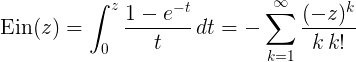Computes the entire complex exponential integral.

Namespace:  Meta.Numerics.Functions
Assembly:  Meta.Numerics (in Meta.Numerics.dll) Version: 4.1.4Syntax
```public static Complex Ein(
Complex z
)```

#### Parameters

z
Type: Meta.NumericsComplex
The complex argument.

#### Return Value

Type: Complex
The value of Ein(z).Remarks

The entire exponential integral function can be defined by an integral or an equivalent series.Both Ei(x) and E1(z) can be obtained from Ein(z).Unlike either Ei(x) or E1(z), Ein(z) is entire, that is, it has no poles or cuts anywhere in the complex plane.See Also